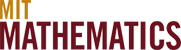# 18.0x

## Lower-Level and Specialty Subjects

Please refer to the Calculus page for comparisons of the various flavors of 18.01 and 18.02. All the versions of these courses are equivalent to each other in their role as prerequisites.

• 18.03 (Differential Equations) is required of Mathematics majors. It has (any flavor of) 18.01 as a prerequisite and (any flavor of) 18.02 as a corequisite. It comes in two variants: 18.03 itself, and 18.032 (formerly 18.034). 18.03 focuses on differential equations for application in science and technology, while 18.032 focuses on differential equations as a mathematical subject and is offered only in the Spring.

• 18.04 (Complex Variables with Applications) has 18.02 and 18.03 as prerequisites. It gives an introduction to the methods of complex analysis, especially as used in applications arising in the physical sciences and engineering. Many Mathematics majors will defer studying complex variables until after they have taken 18.100, and learn about them in 18.112.
• 18.05 (Introduction to Probability and Statistics) Many courses at MIT require acquaintance with probability or statistics, and there are several options for gaining this expertise. The principal ones are 18.05, 6.041, and 18.600. All have 18.02 as a prerequisite. Here is a comparison of these three courses.
• 18.05 is an elementary introduction to both probability and statistics with applications.
• 6.041 is an introduction to probability theory, and modeling and analysis of probabilistic systems, which also includes a treatment of the elements of statistical inference.
• 18.600 covers a broader range of topics in probability, at greater depth than either 18.05 or 6.041, and it is the probability course of choice for most Mathematics majors. 18.650 is a companion course on statistics, accepting either 18.600 or 6.041 as prerequisite.
• 18.06 (Linear Algebra) Another extremely useful mathematical discipline is linear algebra. The Mathematics Department offers several courses on this subject.
• 18.06, listing 18.02 as prerequisite, is a basic subject on matrix theory and linear algebra, emphasizing topics useful in other disciplines. Compared with 18.700, there is more emphasis on matrix algorithms and many applications.
• 18.700 also lists 18.02 as prerequisite. It is a rigorous treatment of linear algebra. Compared with 18.06, there is more emphasis on theory and proofs.
• 18.701 also covers linear algebra, but at a much higher level of abstraction and includes other advanced topics such as group theory.
• 18.062J/6.042J (Mathematics for Computer Science) covers elementary discrete mathematics for computer science and engineering. This subject requires 18.01, and serves as a prerequisite for the basic algorithm subjects, 18.400J/6.045J and 18.404J/6.840J.
• 18.095 (Mathematics Lecture Series) consists of ten lectures by mathematics faculty members on interesting topics from both classical and modern mathematics. It is given over IAP and requires only 18.01.